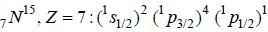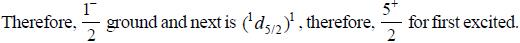Courses

# Test: Nuclear & Particle Physics - 2

## 20 Questions MCQ Test GATE Physics Mock Test Series | Test: Nuclear & Particle Physics - 2

Description
This mock test of Test: Nuclear & Particle Physics - 2 for GATE helps you for every GATE entrance exam. This contains 20 Multiple Choice Questions for GATE Test: Nuclear & Particle Physics - 2 (mcq) to study with solutions a complete question bank. The solved questions answers in this Test: Nuclear & Particle Physics - 2 quiz give you a good mix of easy questions and tough questions. GATE students definitely take this Test: Nuclear & Particle Physics - 2 exercise for a better result in the exam. You can find other Test: Nuclear & Particle Physics - 2 extra questions, long questions & short questions for GATE on EduRev as well by searching above.
*Answer can only contain numeric values
QUESTION: 1

### From semi-emprical mass formula, binding energy is given by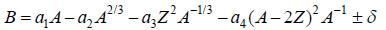If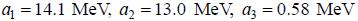and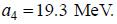The value of Z for most stable nuclei for A = 43 is ____________________( an integer)

Solution: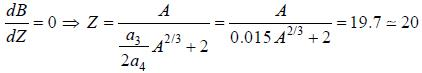*Answer can only contain numeric values
QUESTION: 2

### The meson theory of nuclear forces assumes the virtual exchange ofpions. If a nucleon emits a virtual pion of rest mass 270 me, where me is rest mass of electron), the range of nuclear forces is________fm. (Upto two decimal places)

Solution: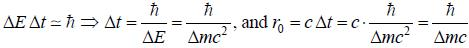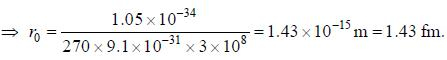QUESTION: 3

### The spins and parities of ground state of 19k39 and 42Mo95  nucleus are respectively

Solution: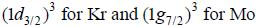QUESTION: 4

Hie magnetic dipole moment and electric qiiadrupole moment for around state of 29Cu63 in μN ( Nuclear masneton units) and bam respectively are

Solution: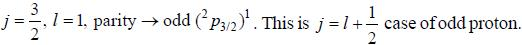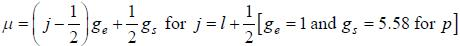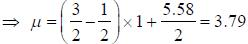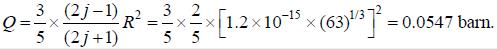QUESTION: 5

What are the expected types of gamma ray transition between tlie following states of odd A nuclei
(1) g9/→ p1/2
(2) h11/→ d3/2

Solution: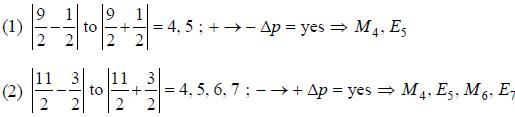QUESTION: 6

(I) If a particle X has Isospin I = 3/2, Baryon no. B = 1 and Strangeness no. S = 0 . then the possible values of electric charges for X are +2, -1, 0 and -1.
(II) If the reaction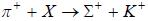is governed by strong interaction then the third component of Isos- pin. Baryon number and Strangeness number ofXare respectively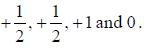(III) The quark content of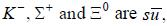uus and uss respectively.
Choose the correct option from the following ?

Solution:

(I) For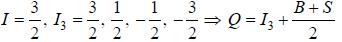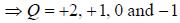(II) Particle X is proton having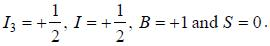(III)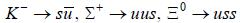QUESTION: 7

For a given nuclear reactions, choose the correct option.
(I)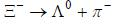(II)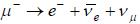(III)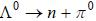(IV)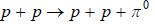(V)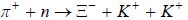Solution:

(I), (II) and (III) are weak and (IV) and (V) are strong.

QUESTION: 8

The reaction 38Sr89 → 39Y89 + β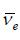undergoes a first forbidden β - decay by

Solution: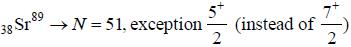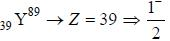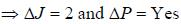Therefore, first forbidden by Gamow-Teller only.

QUESTION: 9

Match tlie following reactions on the left with the associated interactions on file right.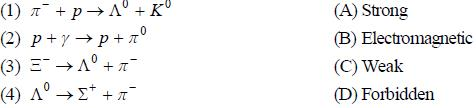Solution:

(1) associated production of strangeness panicles ⇒ strong.
(2) photon involved ⇒ EM.
(3) decay of strange particle ⇒ weak.
(4) light particle cannot decay into heavier ⇒ forbidden.

QUESTION: 10

If the reactions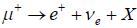and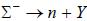are governed by weak interactions, then the particle Z in the reaction Y→ Z + X is

Solution: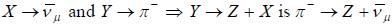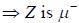*Answer can only contain numeric values
QUESTION: 11

If the first excited rotational state of a nucleus has energy equal to 93.3 KeV then the energy ofthe third excited rotational state is approximately equal to______________KeV. (an integer)

Solution: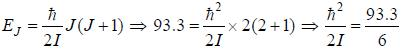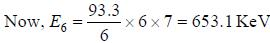*Answer can only contain numeric values
QUESTION: 12

For the reaction P + 1H3 → 1H2 + 1H2 , if T1 is threshold kinetic energy of1 H3 when P is target and T2 is threshold kinetic energy of P when 1H3 is target, then the value of T1- T2 is equal to    MeV. (upto two decimal places)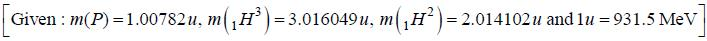Solution: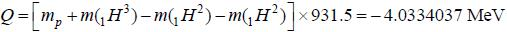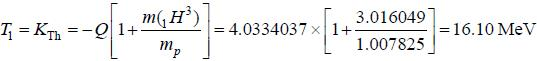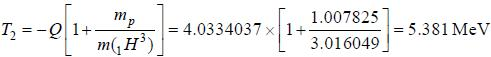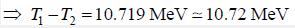*Answer can only contain numeric values
QUESTION: 13

A reactor is developing energy at a rate of 3000 kWatt. If on an average, energy of 200 MeV is released per
fission, then tlie weight of U235 used in 1000 hours of operation is___________gm

Solution:

Energy released per fission = 200 MeV
= 200 x 1.6 x 10-13
= 32 x 10-12 Joule.
Total energy released per second = 3000 kW = 3 x 106 Joule/kW
Number of atoms or fission undergoing per second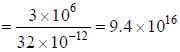Number of atoms undergoing fissions in 1000 hours
= 9.4 x 1016 x 1000 x 60 x 60 = 3.384x 1023
Now, 6.02 x 1023 atoms of U235 weights = 235 gm
Therefore, 3.384 x 1023 atoms o f U235 weights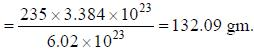*Answer can only contain numeric values
QUESTION: 14

In a reaction 13AI25 → 12Mg25 + e+ + ve , the atomic masses are given as
mA1 = 19.003576u , mMg = 24 .985839u.
The maximum kinetic energy of the neutrino emitted is___________MeV. (upto two decimal places)

Solution: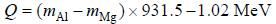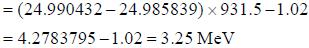*Answer can only contain numeric values
QUESTION: 15

If 86Rn222 nucleus decays at rest by the reaction 86Rn222 → 84Po218 + 2He4  then the kinetic energy of the 84Po218 nucleus is ________________ MeV. (Upto two decimal places)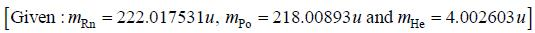Solution: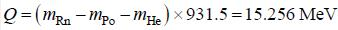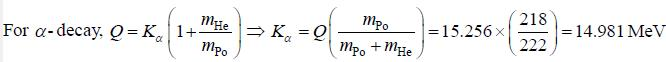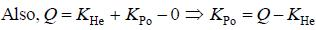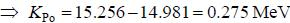QUESTION: 16

If the reaction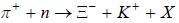is governed by strong interaction, then the particle X is

Solution: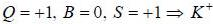QUESTION: 17

Consider the following statements:
P. In reaction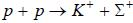, Baryon number, Isospin and third component of Isospin are notcon served.
Q. The reaction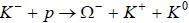proceed via strong interaction.
R. In reaction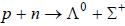, Strangeness number and third component of Isospin are not conserved.
S. The reaction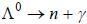proceed via electromagnetic interaction.
Choose the correct option from the following ?

Solution: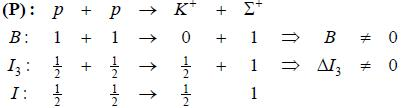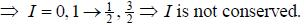Thus, P is correct.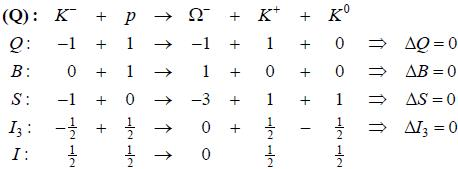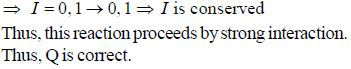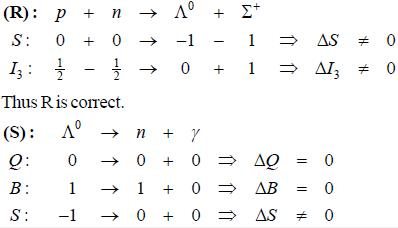*Answer can only contain numeric values
QUESTION: 18

The half life of radon is 3.82 days. If the sample contains initially 2.71 x 1015 atoms, the number of atoms that will disintegrate during die first day are___________x 1014 (upto first decimal places)

Solution: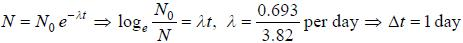The number of undecay atom after one day is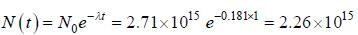Therefore, the number of atom disintergrate during the first day are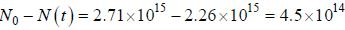*Answer can only contain numeric values
QUESTION: 19

Deutxon in a cyclotron describe a circle of radius 0.32 m just before emerging out of the cyclotron. The frequency of cyclotron is 10 MHz. Mass ofdeutron is 3.32 x 10-27 kg and charge is 1.6 x 10-19 coulomb. The velocity of die deuterons emerging out of the cyclotron is____________107 m/s. (an integer)

Solution: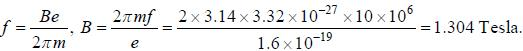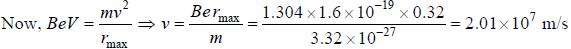QUESTION: 20

According to shell model, the ground state and first excited state spin-parity JP and 7N15 are respectively.

Solution: